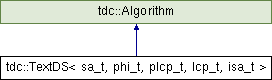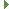tudocomp – The TU Dortmund Compression Framework
tdc::TextDS< sa_t, phi_t, plcp_t, lcp_t, isa_t > Class Template Reference

Manages text related data structures. More...

`#include <TextDS.hpp>`

Inheritance diagram for tdc::TextDS< sa_t, phi_t, plcp_t, lcp_t, isa_t >:## Public Types

using dsflags_t = ds::dsflags_t

using value_type = uliteral_t

using sa_type = sa_t

using phi_type = phi_t

using plcp_type = plcp_t

using lcp_type = lcp_t

using isa_type = isa_t

## Public Member Functions

TextDS (Env &&env, const View &text)

TextDS (Env &&env, const View &text, dsflags_t flags, CompressMode cm=CompressMode::select)

const sa_t & require_sa (CompressMode cm=CompressMode::select)

const phi_t & require_phi (CompressMode cm=CompressMode::select)

const plcp_t & require_plcp (CompressMode cm=CompressMode::select)

const lcp_t & require_lcp (CompressMode cm=CompressMode::select)

const isa_t & require_isa (CompressMode cm=CompressMode::select)

sa_t::data_type inplace_sa (CompressMode cm=CompressMode::select)

phi_t::data_type inplace_phi (CompressMode cm=CompressMode::select)

plcp_t::data_type inplace_plcp (CompressMode cm=CompressMode::select)

lcp_t::data_type inplace_lcp (CompressMode cm=CompressMode::select)

isa_t::data_type inplace_isa (CompressMode cm=CompressMode::select)

sa_t release_sa ()

phi_t release_phi ()

plcp_t release_plcp ()

lcp_t release_lcp ()

isa_t release_isa ()

void require (dsflags_t flags, CompressMode cm=CompressMode::select)

value_type operator[] (size_t i) const
Accesses the input text at position i. More...

const value_typetext () const

size_t size () const
Returns the size of the input text. More...

void print (std::ostream &out, size_t base)Public Member Functions inherited from tdc::Algorithm
virtual ~Algorithm ()=default

Algorithm (Algorithm const &)=default

Algorithm (Algorithm &&)=default

Algorithmoperator= (Algorithm const &)=default

Algorithmoperator= (Algorithm &&)=default

Algorithm (Env &&env)
Instantiates an algorithm in the specified environment. More...

Envenv ()

const Envenv () const

## Static Public Member Functions

static ds::InputRestrictions common_restrictions (dsflags_t flags)

static Meta meta ()

## Static Public Attributes

static const dsflags_t SA = ds::SA

static const dsflags_t ISA = ds::ISA

static const dsflags_t LCP = ds::LCP

static const dsflags_t PHI = ds::PHI

static const dsflags_t PLCP = ds::PLCP

## Detailed Description

### template<typename sa_t = SADivSufSort, typename phi_t = PhiFromSA, typename plcp_t = PLCPFromPhi, typename lcp_t = LCPFromPLCP, typename isa_t = ISAFromSA> class tdc::TextDS< sa_t, phi_t, plcp_t, lcp_t, isa_t >

Manages text related data structures.

Definition at line 30 of file TextDS.hpp.

## ◆ dsflags_t

template<typename sa_t = SADivSufSort, typename phi_t = PhiFromSA, typename plcp_t = PLCPFromPhi, typename lcp_t = LCPFromPLCP, typename isa_t = ISAFromSA>
 using tdc::TextDS< sa_t, phi_t, plcp_t, lcp_t, isa_t >::dsflags_t = ds::dsflags_t

Definition at line 32 of file TextDS.hpp.

## ◆ isa_type

template<typename sa_t = SADivSufSort, typename phi_t = PhiFromSA, typename plcp_t = PLCPFromPhi, typename lcp_t = LCPFromPLCP, typename isa_t = ISAFromSA>
 using tdc::TextDS< sa_t, phi_t, plcp_t, lcp_t, isa_t >::isa_type = isa_t

Definition at line 45 of file TextDS.hpp.

## ◆ lcp_type

template<typename sa_t = SADivSufSort, typename phi_t = PhiFromSA, typename plcp_t = PLCPFromPhi, typename lcp_t = LCPFromPLCP, typename isa_t = ISAFromSA>
 using tdc::TextDS< sa_t, phi_t, plcp_t, lcp_t, isa_t >::lcp_type = lcp_t

Definition at line 44 of file TextDS.hpp.

## ◆ phi_type

template<typename sa_t = SADivSufSort, typename phi_t = PhiFromSA, typename plcp_t = PLCPFromPhi, typename lcp_t = LCPFromPLCP, typename isa_t = ISAFromSA>
 using tdc::TextDS< sa_t, phi_t, plcp_t, lcp_t, isa_t >::phi_type = phi_t

Definition at line 42 of file TextDS.hpp.

## ◆ plcp_type

template<typename sa_t = SADivSufSort, typename phi_t = PhiFromSA, typename plcp_t = PLCPFromPhi, typename lcp_t = LCPFromPLCP, typename isa_t = ISAFromSA>
 using tdc::TextDS< sa_t, phi_t, plcp_t, lcp_t, isa_t >::plcp_type = plcp_t

Definition at line 43 of file TextDS.hpp.

## ◆ sa_type

template<typename sa_t = SADivSufSort, typename phi_t = PhiFromSA, typename plcp_t = PLCPFromPhi, typename lcp_t = LCPFromPLCP, typename isa_t = ISAFromSA>
 using tdc::TextDS< sa_t, phi_t, plcp_t, lcp_t, isa_t >::sa_type = sa_t

Definition at line 41 of file TextDS.hpp.

## ◆ value_type

template<typename sa_t = SADivSufSort, typename phi_t = PhiFromSA, typename plcp_t = PLCPFromPhi, typename lcp_t = LCPFromPLCP, typename isa_t = ISAFromSA>
 using tdc::TextDS< sa_t, phi_t, plcp_t, lcp_t, isa_t >::value_type = uliteral_t

Definition at line 39 of file TextDS.hpp.

## ◆ TextDS() [1/2]

template<typename sa_t = SADivSufSort, typename phi_t = PhiFromSA, typename plcp_t = PLCPFromPhi, typename lcp_t = LCPFromPLCP, typename isa_t = ISAFromSA>
 tdc::TextDS< sa_t, phi_t, plcp_t, lcp_t, isa_t >::TextDS ( Env && env, const View & text )
inline

Definition at line 128 of file TextDS.hpp.

## ◆ TextDS() [2/2]

template<typename sa_t = SADivSufSort, typename phi_t = PhiFromSA, typename plcp_t = PLCPFromPhi, typename lcp_t = LCPFromPLCP, typename isa_t = ISAFromSA>
 tdc::TextDS< sa_t, phi_t, plcp_t, lcp_t, isa_t >::TextDS ( Env && env, const View & text, dsflags_t flags, CompressMode cm = `CompressMode::select` )
inline

Definition at line 150 of file TextDS.hpp.

## ◆ common_restrictions()

template<typename sa_t = SADivSufSort, typename phi_t = PhiFromSA, typename plcp_t = PLCPFromPhi, typename lcp_t = LCPFromPLCP, typename isa_t = ISAFromSA>
 static ds::InputRestrictions tdc::TextDS< sa_t, phi_t, plcp_t, lcp_t, isa_t >::common_restrictions ( dsflags_t flags )
inlinestatic

Definition at line 47 of file TextDS.hpp.

## ◆ inplace_isa()

template<typename sa_t = SADivSufSort, typename phi_t = PhiFromSA, typename plcp_t = PLCPFromPhi, typename lcp_t = LCPFromPLCP, typename isa_t = ISAFromSA>
 isa_t::data_type tdc::TextDS< sa_t, phi_t, plcp_t, lcp_t, isa_t >::inplace_isa ( CompressMode cm = `CompressMode::select` )
inline

Definition at line 196 of file TextDS.hpp.

## ◆ inplace_lcp()

template<typename sa_t = SADivSufSort, typename phi_t = PhiFromSA, typename plcp_t = PLCPFromPhi, typename lcp_t = LCPFromPLCP, typename isa_t = ISAFromSA>
 lcp_t::data_type tdc::TextDS< sa_t, phi_t, plcp_t, lcp_t, isa_t >::inplace_lcp ( CompressMode cm = `CompressMode::select` )
inline

Definition at line 191 of file TextDS.hpp.

## ◆ inplace_phi()

template<typename sa_t = SADivSufSort, typename phi_t = PhiFromSA, typename plcp_t = PLCPFromPhi, typename lcp_t = LCPFromPLCP, typename isa_t = ISAFromSA>
 phi_t::data_type tdc::TextDS< sa_t, phi_t, plcp_t, lcp_t, isa_t >::inplace_phi ( CompressMode cm = `CompressMode::select` )
inline

Definition at line 181 of file TextDS.hpp.

## ◆ inplace_plcp()

template<typename sa_t = SADivSufSort, typename phi_t = PhiFromSA, typename plcp_t = PLCPFromPhi, typename lcp_t = LCPFromPLCP, typename isa_t = ISAFromSA>
 plcp_t::data_type tdc::TextDS< sa_t, phi_t, plcp_t, lcp_t, isa_t >::inplace_plcp ( CompressMode cm = `CompressMode::select` )
inline

Definition at line 186 of file TextDS.hpp.

## ◆ inplace_sa()

template<typename sa_t = SADivSufSort, typename phi_t = PhiFromSA, typename plcp_t = PLCPFromPhi, typename lcp_t = LCPFromPLCP, typename isa_t = ISAFromSA>
 sa_t::data_type tdc::TextDS< sa_t, phi_t, plcp_t, lcp_t, isa_t >::inplace_sa ( CompressMode cm = `CompressMode::select` )
inline

Definition at line 176 of file TextDS.hpp.

## ◆ meta()

template<typename sa_t = SADivSufSort, typename phi_t = PhiFromSA, typename plcp_t = PLCPFromPhi, typename lcp_t = LCPFromPLCP, typename isa_t = ISAFromSA>
 static Meta tdc::TextDS< sa_t, phi_t, plcp_t, lcp_t, isa_t >::meta ( )
inlinestatic

Definition at line 117 of file TextDS.hpp.

## ◆ operator[]()

template<typename sa_t = SADivSufSort, typename phi_t = PhiFromSA, typename plcp_t = PLCPFromPhi, typename lcp_t = LCPFromPLCP, typename isa_t = ISAFromSA>
 value_type tdc::TextDS< sa_t, phi_t, plcp_t, lcp_t, isa_t >::operator[] ( size_t i ) const
inline

Accesses the input text at position i.

Definition at line 295 of file TextDS.hpp.

## ◆ print()

template<typename sa_t = SADivSufSort, typename phi_t = PhiFromSA, typename plcp_t = PLCPFromPhi, typename lcp_t = LCPFromPLCP, typename isa_t = ISAFromSA>
 void tdc::TextDS< sa_t, phi_t, plcp_t, lcp_t, isa_t >::print ( std::ostream & out, size_t base )
inline

Definition at line 309 of file TextDS.hpp.

## ◆ release_isa()

template<typename sa_t = SADivSufSort, typename phi_t = PhiFromSA, typename plcp_t = PLCPFromPhi, typename lcp_t = LCPFromPLCP, typename isa_t = ISAFromSA>
 isa_t tdc::TextDS< sa_t, phi_t, plcp_t, lcp_t, isa_t >::release_isa ( )
inline

Definition at line 216 of file TextDS.hpp.

## ◆ release_lcp()

template<typename sa_t = SADivSufSort, typename phi_t = PhiFromSA, typename plcp_t = PLCPFromPhi, typename lcp_t = LCPFromPLCP, typename isa_t = ISAFromSA>
 lcp_t tdc::TextDS< sa_t, phi_t, plcp_t, lcp_t, isa_t >::release_lcp ( )
inline

Definition at line 213 of file TextDS.hpp.

## ◆ release_phi()

template<typename sa_t = SADivSufSort, typename phi_t = PhiFromSA, typename plcp_t = PLCPFromPhi, typename lcp_t = LCPFromPLCP, typename isa_t = ISAFromSA>
 phi_t tdc::TextDS< sa_t, phi_t, plcp_t, lcp_t, isa_t >::release_phi ( )
inline

Definition at line 207 of file TextDS.hpp.

## ◆ release_plcp()

template<typename sa_t = SADivSufSort, typename phi_t = PhiFromSA, typename plcp_t = PLCPFromPhi, typename lcp_t = LCPFromPLCP, typename isa_t = ISAFromSA>
 plcp_t tdc::TextDS< sa_t, phi_t, plcp_t, lcp_t, isa_t >::release_plcp ( )
inline

Definition at line 210 of file TextDS.hpp.

## ◆ release_sa()

template<typename sa_t = SADivSufSort, typename phi_t = PhiFromSA, typename plcp_t = PLCPFromPhi, typename lcp_t = LCPFromPLCP, typename isa_t = ISAFromSA>
 sa_t tdc::TextDS< sa_t, phi_t, plcp_t, lcp_t, isa_t >::release_sa ( )
inline

Definition at line 204 of file TextDS.hpp.

## ◆ require()

template<typename sa_t = SADivSufSort, typename phi_t = PhiFromSA, typename plcp_t = PLCPFromPhi, typename lcp_t = LCPFromPLCP, typename isa_t = ISAFromSA>
 void tdc::TextDS< sa_t, phi_t, plcp_t, lcp_t, isa_t >::require ( dsflags_t flags, CompressMode cm = `CompressMode::select` )
inline

Definition at line 247 of file TextDS.hpp.

## ◆ require_isa()

template<typename sa_t = SADivSufSort, typename phi_t = PhiFromSA, typename plcp_t = PLCPFromPhi, typename lcp_t = LCPFromPLCP, typename isa_t = ISAFromSA>
 const isa_t& tdc::TextDS< sa_t, phi_t, plcp_t, lcp_t, isa_t >::require_isa ( CompressMode cm = `CompressMode::select` )
inline

Definition at line 170 of file TextDS.hpp.

## ◆ require_lcp()

template<typename sa_t = SADivSufSort, typename phi_t = PhiFromSA, typename plcp_t = PLCPFromPhi, typename lcp_t = LCPFromPLCP, typename isa_t = ISAFromSA>
 const lcp_t& tdc::TextDS< sa_t, phi_t, plcp_t, lcp_t, isa_t >::require_lcp ( CompressMode cm = `CompressMode::select` )
inline

Definition at line 167 of file TextDS.hpp.

## ◆ require_phi()

template<typename sa_t = SADivSufSort, typename phi_t = PhiFromSA, typename plcp_t = PLCPFromPhi, typename lcp_t = LCPFromPLCP, typename isa_t = ISAFromSA>
 const phi_t& tdc::TextDS< sa_t, phi_t, plcp_t, lcp_t, isa_t >::require_phi ( CompressMode cm = `CompressMode::select` )
inline

Definition at line 161 of file TextDS.hpp.

## ◆ require_plcp()

template<typename sa_t = SADivSufSort, typename phi_t = PhiFromSA, typename plcp_t = PLCPFromPhi, typename lcp_t = LCPFromPLCP, typename isa_t = ISAFromSA>
 const plcp_t& tdc::TextDS< sa_t, phi_t, plcp_t, lcp_t, isa_t >::require_plcp ( CompressMode cm = `CompressMode::select` )
inline

Definition at line 164 of file TextDS.hpp.

## ◆ require_sa()

template<typename sa_t = SADivSufSort, typename phi_t = PhiFromSA, typename plcp_t = PLCPFromPhi, typename lcp_t = LCPFromPLCP, typename isa_t = ISAFromSA>
 const sa_t& tdc::TextDS< sa_t, phi_t, plcp_t, lcp_t, isa_t >::require_sa ( CompressMode cm = `CompressMode::select` )
inline

Definition at line 158 of file TextDS.hpp.

## ◆ size()

template<typename sa_t = SADivSufSort, typename phi_t = PhiFromSA, typename plcp_t = PLCPFromPhi, typename lcp_t = LCPFromPLCP, typename isa_t = ISAFromSA>
 size_t tdc::TextDS< sa_t, phi_t, plcp_t, lcp_t, isa_t >::size ( ) const
inline

Returns the size of the input text.

Definition at line 305 of file TextDS.hpp.

## ◆ text()

template<typename sa_t = SADivSufSort, typename phi_t = PhiFromSA, typename plcp_t = PLCPFromPhi, typename lcp_t = LCPFromPLCP, typename isa_t = ISAFromSA>
 const value_type* tdc::TextDS< sa_t, phi_t, plcp_t, lcp_t, isa_t >::text ( ) const
inline

Definition at line 300 of file TextDS.hpp.

## ◆ ISA

template<typename sa_t = SADivSufSort, typename phi_t = PhiFromSA, typename plcp_t = PLCPFromPhi, typename lcp_t = LCPFromPLCP, typename isa_t = ISAFromSA>
 const dsflags_t tdc::TextDS< sa_t, phi_t, plcp_t, lcp_t, isa_t >::ISA = ds::ISA
static

Definition at line 34 of file TextDS.hpp.

## ◆ LCP

template<typename sa_t = SADivSufSort, typename phi_t = PhiFromSA, typename plcp_t = PLCPFromPhi, typename lcp_t = LCPFromPLCP, typename isa_t = ISAFromSA>
 const dsflags_t tdc::TextDS< sa_t, phi_t, plcp_t, lcp_t, isa_t >::LCP = ds::LCP
static

Definition at line 35 of file TextDS.hpp.

## ◆ PHI

template<typename sa_t = SADivSufSort, typename phi_t = PhiFromSA, typename plcp_t = PLCPFromPhi, typename lcp_t = LCPFromPLCP, typename isa_t = ISAFromSA>
 const dsflags_t tdc::TextDS< sa_t, phi_t, plcp_t, lcp_t, isa_t >::PHI = ds::PHI
static

Definition at line 36 of file TextDS.hpp.

## ◆ PLCP

template<typename sa_t = SADivSufSort, typename phi_t = PhiFromSA, typename plcp_t = PLCPFromPhi, typename lcp_t = LCPFromPLCP, typename isa_t = ISAFromSA>
 const dsflags_t tdc::TextDS< sa_t, phi_t, plcp_t, lcp_t, isa_t >::PLCP = ds::PLCP
static

Definition at line 37 of file TextDS.hpp.

## ◆ SA

template<typename sa_t = SADivSufSort, typename phi_t = PhiFromSA, typename plcp_t = PLCPFromPhi, typename lcp_t = LCPFromPLCP, typename isa_t = ISAFromSA>
 const dsflags_t tdc::TextDS< sa_t, phi_t, plcp_t, lcp_t, isa_t >::SA = ds::SA
static

Definition at line 33 of file TextDS.hpp.

The documentation for this class was generated from the following file: